CAT 2022 Profit and Loss: Formulas, Shortcuts and Tricks

The purpose of this article is very simple: to provide you a list of Profit and Loss Formulas, Shortcuts and Tricks that you can use to solve questions. Remember, the purpose here is to just to provide you a handy list of results that you can use. This article does not provide any derivations or detailed concepts for this topic. The first four articles for this topic provide you the same. You the table of contents given below to go through these articles.

1. One can generate a profit only if Selling Price> Cost Price

2. One generates a loss when Selling Price < Cost Price.

3. Profit = Selling Price – Cost Price
%profit = {(Selling Price – Cost Price)/Cost Price} x 100

4. Loss = Cost Price – Selling Price
%Loss = {(Cost Price – Selling Price)/Cost Price} x 100

5. Sale price :- If there is a profit of P %,
Cost Price = C
Then SP = {(100+P)/100}xC

6. If there is a loss of L %,
Cost Price = C
Then
SP = {(100-L)/100}xC

7. Cost price :-
If there is a profit of P %,
Cost Price = C
Sale price= SP
Then C = {100/(100+p)} x SP
If there is a loss of L %,
Then
C = {100/(100-L)}xSP

8. A dishonest dealer claims to sell his goods at cost price ,but he uses a weight of lesser weight .Find his gain%.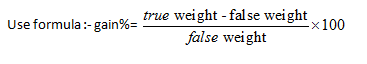9. A shopkeeper sells an item at a profit of x % and uses a weight which is y % less .find his total profit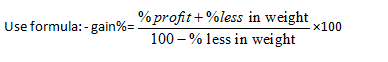10. When dealer sells goods at loss on cost price but uses less weight .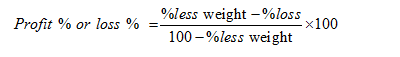11. A dishonest dealer sells goods at x % loss on cost price but uses a gm instead of b gm . his profit or loss percent :-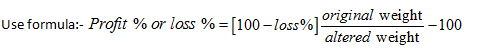Note :- profit or loss will be decided according to sign .if +ive it is profit ,if –ve it is loss .

12.  If the price of an item increases by r%  , then the reduction in consumption so that  expenditure remains the same  ,is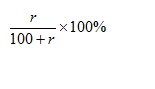13. If the price of a commodity decreases by r% then increase in consumption , so as not to decrease expenditure on this item is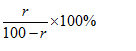14. A reduction of x% in price enables a person to buy y kg more for Rs. A. Then the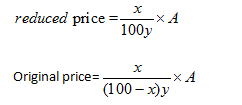15. When there are two successive profits of x% and y% then the net percentage profit =[x+y+xy/100]

When there is a profit of x% and loss of y% then net percentage profit or loss = [x – y – xy/100]

Note: If the final sign in the above expression is positive then there is net profit but if it is negative then there is net loss.

16. A sells goods to B at a profit of x% andB sells it to C at a profit of y%. If C pays RsP for it,then the cost price for A is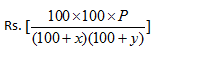Note:- for loss replace plus sign with minus .

17. When each of the two things is sold at the same price,and a profit of p% is made on the first and a loss of L% is made on the second,then the percentage gain or loss is .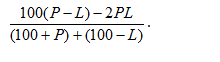18. If profit percentage and loss percentage are equal, put P=L

=>   %loss = p2 /100

19.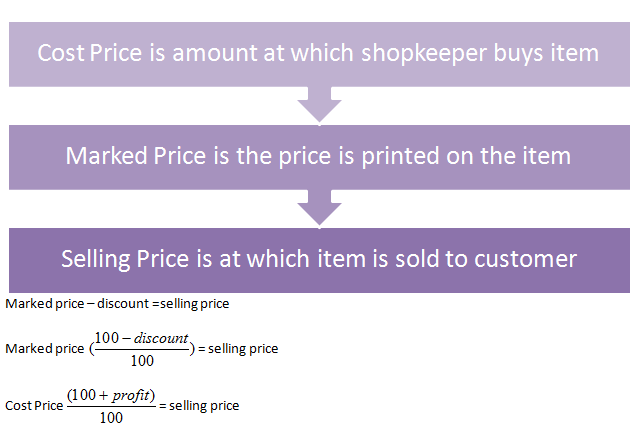This completes this list of Profit and Loss formulas, shortcuts and tricks. Use this as a handy revision guide for this topic.